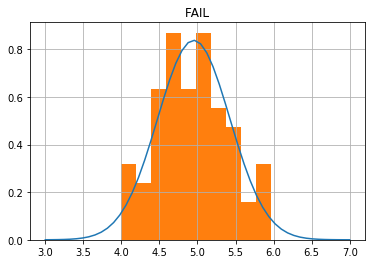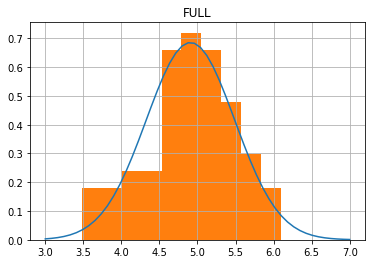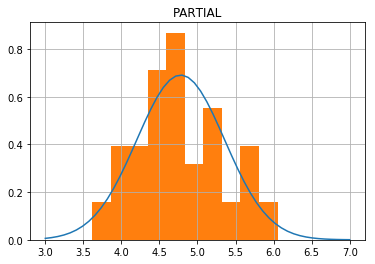# Academic Coding - Wrangling Data Into a Computer

Posted on 22-05-19 in Computing

## Overview¶

One of the more common endeavours of a academic is interpretting data. Today this can mean processing hundreds if not thousands of files produced by a piece of apparatus. Fortunately, most (sadly closed-source) software attached to these tools will output some sort of plain-text. Unfortunately, they are invariably difficult to parse and full of oddities that need to be worked around.

In this first article I will discuss a few ways to open and parse large quantities of such files using Python.

## Getting Started¶

To begin, let's create some mock data.

In :
import csv
from random import randint, choice, random
import datetime
import os

from scipy.stats import norm

def generate_spoof_file(title, date, values=180):
"""
Generate a CSV file holding mock data for us to play with
"""
options = ('FULL','PARTIAL','FAIL')
parameters = [str(randint(-5,5)) for _ in range(5)]
parameters = ",".join(parameters)

with open(title, 'w', newline='') as csvfile:
writer = csv.writer(csvfile, delimiter=";", quotechar="\'")
writer.writerow(['Expensive Apparatus'])
writer.writerow(['Param :: ({})'.format(parameters)])
writer.writerow(['Date :: {}'.format(date)])
writer.writerow([])
writer.writerow(['id','time','alter','value','profile',''])

val = norm(loc=5, scale=0.5).rvs(values)
time = datetime.datetime(year=2019, month=5, day=21, hour=3, minute=11)
for j in range(values):
time += datetime.timedelta(minutes=1)
writer.writerow([j,time.time(),randint(0,100), "{:.5f}".format(val[j]), choice(options)])

def generate_files(loc='input', num_of_files=10):
"""
Generates spoof files in a subdirectory
"""
try:
os.mkdir(loc)
except FileExistsError:

while len(os.listdir(loc)) < num_of_files:
word = choice(('typical', 'important', 'good', 'weird'))
date = "{day}{month}2018".format(day=randint(1,28), month=randint(1,12))
filename = "{}_{}_run{}.csv".format(word, date, choice('AB'))
print("Creating", filename)
location = os.path.join(loc, filename)
generate_spoof_file(location, date=date)

generate_files()

Creating good_2432018_runA.csv
Creating good_362018_runB.csv
Creating important_442018_runA.csv
Creating weird_172018_runB.csv
Creating typical_2812018_runB.csv
Creating good_2132018_runA.csv
Creating typical_1162018_runA.csv
Creating good_942018_runA.csv
Creating good_19122018_runB.csv
Creating important_2112018_runB.csv


Let's look at one file

In :
with open('input/good_2432018_runA.csv') as f:
for j, row in enumerate(f):
if j > 10:
break
print(j, row, end='')

0 Expensive Apparatus
1 Param :: (2,1,0,1,3)
2 Date :: 2432018
3
4 id;time;alter;value;profile;
5 0;03:12:00;34;4.24380;FULL
6 1;03:13:00;71;5.62660;FAIL
7 2;03:14:00;81;5.24813;FAIL
8 3;03:15:00;27;5.04068;PARTIAL
9 4;03:16:00;76;5.47492;FULL
10 5;03:17:00;0;4.27515;FULL


Here we can see that I've introduced many examples of egregious error.

• inconsistent seperators
• parameters stored in the filename and in the preamble
• unclear datatypes

In a magical world, certainly not this one, files will be in a sensible format and will trivially load into a variety of tools. In reality they will be either be optimised for importing into MS Excel or not at all. Luckily for us we can resist the urge to write a DSL (http://blog.erezsh.com/how-to-write-a-dsl-in-python-with-lark/) most of the time.

The first lesson is there is no such thing as a standard CSV file. In many cases files will include arbitrary information, not to mention user edits. Additional information is always needed - where a measurement was taken and with what sample.

Finally before I begin diving into more code, let me say that pandas should be used unless you've been instructed to do so otherwise or you simply cannot get it installed. It will save a significant portion of your sanity not to mention time!

## Getting Filenames¶

Let's write a simple program that will fetch all files with the same extension, csv, along with their full path

In :
import os
root_directory = 'input'
files_to_parse = {}  # filename : path

for root, dirs, files in os.walk(root_directory):
for fn in files:
if fn.endswith('csv'):
files_to_parse[fn] = os.path.join(root, fn)

files_to_parse

Out:
{'good_2132018_runA.csv': 'input/good_2132018_runA.csv',
'typical_1162018_runA.csv': 'input/typical_1162018_runA.csv',
'typical_2812018_runB.csv': 'input/typical_2812018_runB.csv',
'good_362018_runB.csv': 'input/good_362018_runB.csv',
'good_942018_runA.csv': 'input/good_942018_runA.csv',
'important_442018_runA.csv': 'input/important_442018_runA.csv',
'good_2432018_runA.csv': 'input/good_2432018_runA.csv',
'weird_172018_runB.csv': 'input/weird_172018_runB.csv',
'important_2112018_runB.csv': 'input/important_2112018_runB.csv',
'good_19122018_runB.csv': 'input/good_19122018_runB.csv'}

We can use this dictionary to load files into Python

In :
line_seperator = ';'

for filename in files_to_parse:
print(f'Parsing : {filename}')
with open(files_to_parse[filename]) as f:
for j, line in enumerate(f):
line = line.rstrip('\r\n')  # get rid of tabs and newlines at end of rows
if j > 3:
line = line.split(line_seperator)
line = [itm for itm in line if itm]  # get rid of empty spaces

print(j, line)
if j > 10:
break
break

Parsing : good_2132018_runA.csv
0 Expensive Apparatus
1 Param :: (0,5,5,-4,2)
2 Date :: 2132018
3
4 ['id', 'time', 'alter', 'value', 'profile']
5 ['0', '03:12:00', '24', '4.09662', 'PARTIAL']
6 ['1', '03:13:00', '3', '4.86562', 'FAIL']
7 ['2', '03:14:00', '87', '4.55483', 'FAIL']
8 ['3', '03:15:00', '47', '5.29659', 'FULL']
9 ['4', '03:16:00', '87', '4.11432', 'PARTIAL']
10 ['5', '03:17:00', '42', '3.48613', 'FULL']
11 ['6', '03:18:00', '17', '4.72914', 'PARTIAL']


Here we can see the row headers are given to us.

['id', 'time', 'alter', 'value', 'profile']



Let's define a dictionary containing the datatypes for each and use this to parse our data. We'll also tidy this up a bit.

In :
from dateutil import parser

def parse_datetime(time):
return parser.parse(time).time()

def parse_file(location, line_seperator = ';', **parameters):
'''
Parse file using information discussed

'''
_rows = {'id' : int, 'time' :  parse_datetime, 'alter' : int, 'value' : float, 'profile' : str}
rows = parameters.get('rows', _rows)

with open(location) as f:
for j, line in enumerate(f):
line = line.rstrip('\r\n')  # get rid of tabs and newlines at end of rows
if j > 4:
line = line.split(line_seperator)
line = [itm for itm in line if itm]  # get rid of empty spaces
line = {lbl : rows[lbl](value) for value, lbl in zip(line, rows.keys())}
yield line

loaded_data = {filename : list(parse_file(files_to_parse[filename])) for filename in files_to_parse}

In :
fn = 'good_2132018_runA.csv'
print(f'Data from {fn}')
print(j, row)

if j >= 10:
break

Data from good_2132018_runA.csv
0 {'id': 0, 'time': datetime.time(3, 12), 'alter': 24, 'value': 4.09662, 'profile': 'PARTIAL'}
1 {'id': 1, 'time': datetime.time(3, 13), 'alter': 3, 'value': 4.86562, 'profile': 'FAIL'}
2 {'id': 2, 'time': datetime.time(3, 14), 'alter': 87, 'value': 4.55483, 'profile': 'FAIL'}
3 {'id': 3, 'time': datetime.time(3, 15), 'alter': 47, 'value': 5.29659, 'profile': 'FULL'}
4 {'id': 4, 'time': datetime.time(3, 16), 'alter': 87, 'value': 4.11432, 'profile': 'PARTIAL'}
5 {'id': 5, 'time': datetime.time(3, 17), 'alter': 42, 'value': 3.48613, 'profile': 'FULL'}
6 {'id': 6, 'time': datetime.time(3, 18), 'alter': 17, 'value': 4.72914, 'profile': 'PARTIAL'}
7 {'id': 7, 'time': datetime.time(3, 19), 'alter': 43, 'value': 5.75434, 'profile': 'FULL'}
8 {'id': 8, 'time': datetime.time(3, 20), 'alter': 82, 'value': 5.94915, 'profile': 'FAIL'}
9 {'id': 9, 'time': datetime.time(3, 21), 'alter': 7, 'value': 4.89036, 'profile': 'FULL'}
10 {'id': 10, 'time': datetime.time(3, 22), 'alter': 77, 'value': 4.23641, 'profile': 'FULL'}


From this stage it will come down to what we wish to calculate. Let's look at grouping data by PROFILE and see what the mean and standard deviation of value is.

In :
from statistics import mean, stdev

In :
for filename in loaded_data:
for profile in ('FAIL', 'FULL', 'PARTIAL'):
data = [row['value'] for row in loaded_data[filename] if row['profile'] == profile]
mean_value = mean(data)
std_value = stdev(data)
print(f"{filename}, {profile} has a mean of {mean_value:.2f} +/- {std_value:.2f}")
break  # only prints first filename!

good_2132018_runA.csv, FAIL has a mean of 4.95 +/- 0.48
good_2132018_runA.csv, FULL has a mean of 4.91 +/- 0.59
good_2132018_runA.csv, PARTIAL has a mean of 4.78 +/- 0.58


This approach is probably the most common, however the mess of dictionaries and iteration will start to annoy you if you are forced to continue expanding this over and over again.

Furthermore this approach is assuming each file is well-defined. In reality this approach is only set by examiners and in cases when using external Python libraries is not advisable. As this is not sane, let us look at a better approach :

## Libraries - Why you should be using Pandas¶

Ultimately we should avoid using the above approach simply because it gets in the way of the actual job at hand - processing our data. The simplest approach will be to using the pandas library like so

In :
import pandas as pd

filename = 'good_2132018_runA.csv'
location = files_to_parse[filename]

df = pd.read_csv(location, index_col=0, skiprows=5, sep=';', parse_dates=,
names=('id', 'time', 'alter', 'value', 'profile'),
)

df.time = df.time.apply(lambda row : row.time())  # get rid of date bit


Out:
time alter value profile
id
0 03:12:00 24 4.09662 PARTIAL
1 03:13:00 3 4.86562 FAIL
2 03:14:00 87 4.55483 FAIL
3 03:15:00 47 5.29659 FULL
4 03:16:00 87 4.11432 PARTIAL

Here pandas has done a great deal of the legwork for us.

Ultimately we do not want to waste our time on reinventing the wheel

Let's repeat the job of grouping by profile and this time let's calculate a few more statistics

In :
filename = 'good_2132018_runA.csv'

for profile, grp in df.groupby('profile'):
mean_value = grp.value.mean()
std_value = grp.value.std()
print(f"{filename}, {profile} has a mean of {mean_value:.2f} +/- {std_value:.2f}")

good_2132018_runA.csv, FAIL has a mean of 4.95 +/- 0.48
good_2132018_runA.csv, FULL has a mean of 4.91 +/- 0.59
good_2132018_runA.csv, PARTIAL has a mean of 4.78 +/- 0.58


As an example let's use Pandas and a graphing library, matplotlib to look at the distribution of our data directly.

In :
from scipy.stats import norm
from matplotlib.pyplot import subplots, show
from numpy import linspace

x = linspace(3, 7)
for profile, grp in df.groupby('profile'):

loc, scale = norm.fit(grp.value)
print(f'{loc:.2f} +/- {scale:.2f}')

fig, ax = subplots()
ax.set_title(profile)

ax.plot(x, norm.pdf(x, loc=loc, scale=scale))
data = grp.value.hist(density=1,ax=ax)

show()

4.95 +/- 0.484.91 +/- 0.584.78 +/- 0.58This was a very minor beginning. I've glossed over the most dangerous aspect of academic coding - lack of testing. With no verification of your analysis or code, it is far too easy to let mistakes slip in.

In the next post we will explore testing methodologies and how these can be used to increase the reliability of our analysis.